# How to Sum by Group in Excel

Sometimes we may have the requirement that subtotal data by group in a table. To just subtotal data in a column, we can use SUM function directly; for data in a column, amount data in another column, we can use SUMIF function to subtotal data. But to subtotal data in group, we need to classify data by group firstly, then subtotal data which belongs to the same group. Above all, to subtotal data by group, we need to use formula based on IF function and SUMIF function. IF function is used for confirm ‘if value in a cell equals to its cell above’, if yes, then SUMIF function will be triggered and returns the corresponding total amount. This tutorial will help you to know the method with simple description, screenshots and explanation clearly. You can refer to below example for understanding well.

See example below: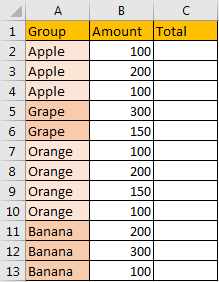You can see that fruits are classified to different groups, if you want to subtotal data by group, do you know the proper formula in this instance?

In C2, enter the formula =IF(A2=A1,” “,SUMIF(\$A\$2:\$A\$13,A2,\$B\$2:\$B\$13)).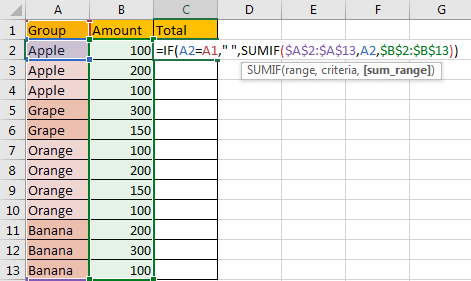In this instance, we use IF function to return the subtotal value. We know that for IF function, =IF(logical_test, [value if true], [value if false]). In this formula, we use A2=A1 to classify data, if the two values are the same, nothing will be returned due to they belong to the same group, if they are different, that means a new group is detected, the SUMIF function will be used here.

And for SUMIF function =SUMIF(range, criteria, [sum range]), in this instance, we need to subtotal data by group, \$A\$2:\$A\$3 is an absolute range for criteria, each data in column A is a criteria, \$B\$2:\$B\$3 is an absolute range for matching values, so if we enter SUM(\$A\$2:\$A\$3,A2, \$B\$2:\$B\$3) here, excel will search A2 value ‘Apple’ in \$A\$2:\$A\$3, and find all ‘Apple’ in this range, then subtotal all matching values in \$B\$2:\$B\$3, then return SUMIF value in C2 finally.

Click Enter to get returned value. We can see that 400 is correct.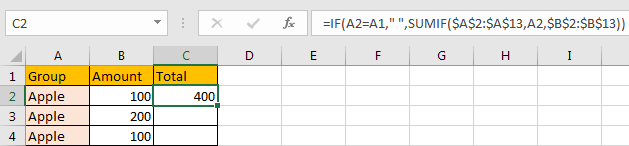Drag down the cell to copy formula. We can see that subtotal is recorded in the first row of each group. That’s because in this row, the data in column A is different with its cell above, a new group is found.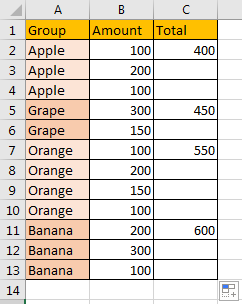Notes:

1. In this instance, it is easy to locate the beginning data and ending data in a column, if there are multiple rows in the table and you are hard to find the ending data, you can use the full column as reference. Then formula will update to =IF(A2=A1,” “,SUMIF(A:A,A2,B:B)), it does work.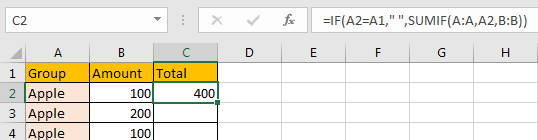2. If you want to record subtotal in the last tow of each group, just enter the formula in the last row of the first group, and copy cell for the following cells.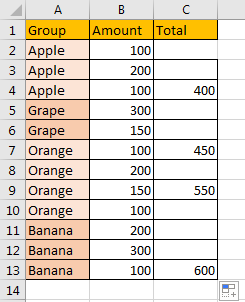### Related Functions

• Excel SUMIF Function
The Excel SUMIF function sum the numbers in the range of cells that meet a single criteria that you specify. The syntax of the SUMIF function is as below:=SUMIF (range, criteria, [sum_range])…
• Excel SUM function
The Excel SUM function will adds all numbers in a range of cells and returns the sum of these values. You can add individual values, cell references or ranges in excel.The syntax of the SUM function is as below:= SUM(number1,[number2],…)…
• Excel IF function
The Excel IF function perform a logical test to return one value if the condition is TRUE and return another value if the condition is FALSE. The IF function is a build-in function in Microsoft Excel and it is categorized as a Logical Function.The syntax of the IF function is as below:= IF (condition, [true_value], [false_value])….